# Machine Learning Regression Adjustments in A/B Tests

##### Background

A/B testing is the gold standard when interested in measuring causal relationships with data. In settings with small treatment effects or underpowered designs, a major focus falls on decreasing the variance. In simple low-dimensional settings a common attempt to do that is to include a bunch of covariates and their interaction with the treatment variable in an OLS regression. Under standard assumptions, the coefficient on the treatment variable is still asymptotically unbiased (albeit not in finite samples) and including the interactions guarantees that this estimator does not have higher asymptotic variance than the simple difference-in-means.

In high-dimensional settings, however, this can easily lead to overfitting and new tools for variance reduction are needed. In this article, I will focus on two ways Machine Learning (ML) can be helpful with this problem when we have access to a bunch of covariates.  In the first set of methods, we use a ML algorithm (such as the lasso) to directly estimate the treatment effect. Alternatively, we can first use ML to predict the outcome and then feed that prediction in an OLS regression.

A helpful benchmark with which to compare these methods is the simple (non-parametric) difference-in-means estimator. Under certain conditions, both approaches guarantee smaller or equal variance.

So, let’s get started.

The notation is as follows. I use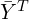and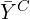to denote the sample average outcomes for the treatment and control groups respectively.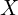is the covariate vector and its deviations from the average are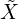. The benchmark estimator can be expressed as: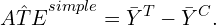##### Two ML Methods for Variance Reduction
###### Use ML Regression Directly

The simplest and most natural way to incorporate covariates is to add them to a linear model (along with the treatment variable and their interactions with the treatment variable). Bloniarz et al. (2015) show we can directly use Lasso regression instead of OLS.

To guarantee that the lasso does not omit the treatment variable, we can run two separate regressions, one for each (treatment) group. Then the estimator can be formulated as: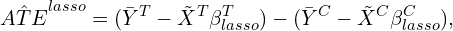where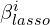is the coefficient vector from the lasso regressions on observations in group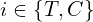. The authors also give a conservative formula for computing the variance of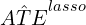. When the two lasso regressions select different sets of covariates (which is probably common in practice), this is no longer guaranteed to yield equal or lower asymptotic variance compared to the benchmark.

Algorithm:

• For the treatment and control groups separately, run lasso regression of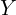ongo get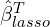and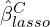.
• Calculate the treatment effect estimateusing the above formula.
• Calculate the estimate of the variance ofusing the formula in Blonarz et al. (2015).

The authors also propose the lasso+OLS estimator which first uses L1 regularization as above to select the covariates and then plugs those in OLS to get the treatment effect estimate.

A similar idea has also been studied by Wager et al (2016). They show that when additionally, assuming Gaussian data (along with a bunch of regularity assumptions), we can use any “risk consistent” ML estimator such as ridge, elastic net, etc. “Risk consistent” here means as we give the algorithm more data, it gets closer to the truth. The lower the risk the higher the variance reduction gains compared to the simple difference-in-means estimator. The authors also propose a simple cross-fitting approach to calculate confidence intervals.

Algorithm:

• Split the data into k equal sized folds.
• For each fold k:
• calculate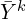,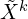.
• get the coefficients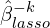based on regressions on all other k-1 folds.
• combine both quantities and calculate.
• calculate its standard error.
• Get the final estimatesand its standard error by taking weighted averages across all k folds.

This concludes the discussion of using a ML-type linear regression model to reduce the variance in A/B tests. Let’s now move on to the second method.

###### Use ML Regression Indirectly

An alternative approach first uses ML to predictand then plugs that prediction into an OLS regression of the outcome on the treatment variable. One can then use cross-fitting to do the prediction which ensures the “naïve” OLS confidence intervals remain valid. The authors call this procedure MLRATE (machine learning regression-adjusted treatment effect estimator).

Here is a rough version of the algorithm:

Algorithm:

• Split the data in k equal-sized folds.
• For each fold k:
• Predictby applying a ML algorithm to all other k-1 folds. Call this prediction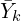.
• Get a final prediction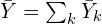.
• Run OLS ofon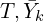and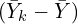and use the associated standard errors and p-values.
##### Bottom Line
• Regression adjustments are a commonly used tool to reduce variance in A/B tests.
• The machine learning toolbox offers possibly more powerful algorithms in this space.
• There are two main ML approaches. Both can be shown under certain conditions to be at least as good as the simple difference-in-means estimator.
• The first approach uses ML regression algorithms directly.
• The second method, instead, uses ML to predict the outcome and adds that in an OLS regression.

Unfortunately, I do not know of any papers that summarize or compare these methods. If you are interested in learning more, look at the original references and any papers mentioned therein.

##### References

Belloni, A., & Chernozhukov, V. (2013). Least squares after model selection in high-dimensional sparse models. Bernoulli 19(2): 521-547

Bloniarz, A., Liu, H., Zhang, C. H., Sekhon, J. S., & Yu, B. (2016). Lasso adjustments of treatment effect estimates in randomized experiments. Proceedings of the National Academy of Sciences, 113(27), 7383-7390.

Guo, Y., Coey, D., Konutgan, M., Li, W., Schoener, C., & Goldman, M. (2021). Machine learning for variance reduction in online experiments. Advances in Neural Information Processing Systems, 34, 8637-8648.

Lin, W. (2013). Agnostic notes on regression adjustments to experimental data: Reexamining Freedman’s critique. Ann. Appl. Stat. 7(1): 295-318

List, J. A., Muir, I., & Sun, G. K. (2022). Using Machine Learning for Efficient Flexible Regression Adjustment in Economic Experiments (No. w30756). National Bureau of Economic Research.

Negi, A., & Wooldridge, J. M. (2021). Revisiting regression adjustment in experiments with heterogeneous treatment effects. Econometric Reviews, 40(5), 504-534.

Poyarkov, A., Drutsa, A., Khalyavin, A., Gusev, G., & Serdyukov, P. (2016, August). Boosted decision tree regression adjustment for variance reduction in online controlled experiments. In Proceedings of the 22nd ACM SIGKDD International Conference on Knowledge Discovery and Data Mining (pp. 235-244).

Wager, S., Du, W., Taylor, J., & Tibshirani, R. J. (2016). High-dimensional regression adjustments in randomized experiments. Proceedings of the National Academy of Sciences, 113(45), 12673-12678.